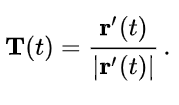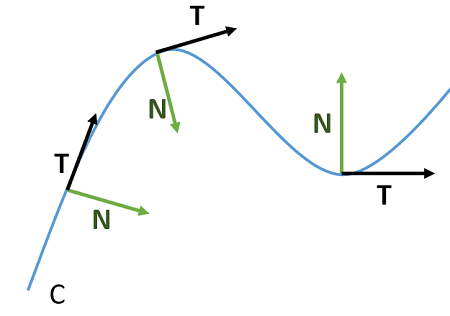# Tangent Vector (Velocity Vector)

A tangent vector T (also called a velocity vector) shows the direction of motion. It points in the direction of the tangent line and has its base at the point of tangency on the curve rather than the origin. They are often used to study bends on a curve, because bends are a result of the change in direction.

## Unit Tangent Vector Definition

The unit tangent vector is defined asWhere r′(t) is the derivative of the position vector.

The unit tangent vector and unit normal vector are at right angles to each other on a curve. Unit tangent vectors “fly off” a curve like a roller coaster car off its tracks, while unit normal vectors always point to the “inside” of a curve:A smooth curve has a tangent vector at every point.

## How to Find the Unit Tangent Vector

Example question: Find the unit tangent vector for the function components
r(t) = <t, 3cost, 3sint>.

Step 1: Take the derivatives of the components. We have three components, so we’ll need to find three derivatives:

 Component Derivative t 1 3cost -3sint 3sint 3cost

This gives us the numerator of the tangent vector formula:
r′(t) = <1, -3sint, 3 cost>

Step 2 Find the Magnitude of r′(t) from Step 1. This is the denominator in the tangent vector formula.

1. Square each of the components:
• 12 = 1
• 3sint2 = 9sin2t
• 3 cost>2 = 9cos2t
2. Add the squared components together: 1 + 9sin2t + 9cos2t
3. Take the square root: √ ( 1 + 9sin2t + 9cos2t)
4. Simplify by factoring out a 9: √ ( 1 + 9(sin2t + cos2t))
5. Substitute using the trigonometric identity sin2t + cos2t = 1, which leaves us with √(10).

Step 3Insert the values from Steps 1 and 2 into the formula:CITE THIS AS:
Stephanie Glen. "Tangent Vector (Velocity Vector)" From StatisticsHowTo.com: Elementary Statistics for the rest of us! https://www.statisticshowto.com/tangent-vector-velocity/